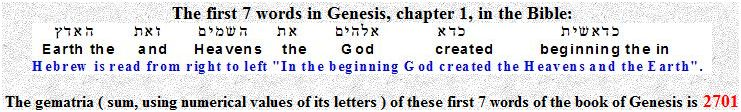Home | Study Links | Poems |

## Alpha ( α )The famous quantity alpha (α), the so-called the fine-structure constant is currently measured to:
1/137.03599976 = 0.007297352533

Three factual numbers, π 3.141…, 7 and 2701 combined, form a simple equation approximately equal to alpha:

pi (π) = 3.1415926535...

7 is the number of Spiritual perfection

Andπ² x 7 x 2701 = 186604.610...mps

√186604.610...mps = 431.977.../π = 137.502727245680476357...2 = 18907 (7 x 2701)

1/137.502727245680476357... = .007272583024577165... =Perhaps one day, the fine structure value 0.007297352533..., can be expressed as a simple equation such as this:##### “We know what kind of a dance to do experimentally to measure this number 0.00729...very accurately, but we don't know what kind of dance to do on the computer to make this number come out, without putting it in secretly!”Richard P. Feynman

<><

1+8+9+0+7= 25
5x2= 10(1+0)= --
1
5-2= ------------3
5+2= ------------7
137